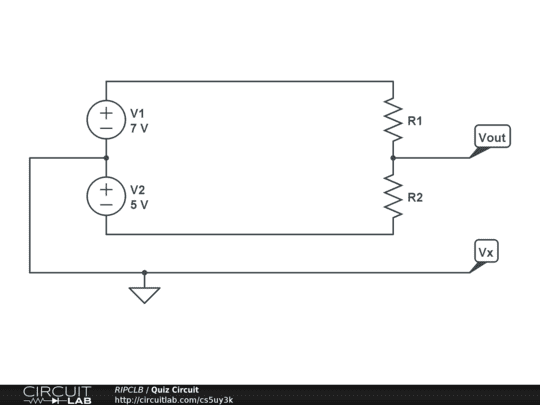# Voltage With Respect to Ground

RIPCLB

## Homework Statement

I have the following circuit:The problem states to give arbitrary values to the resistors, and to find the voltage at Vout with respect to the ground, which I named Vx.

## The Attempt at a Solution

I assume that the voltage at Vx is going to be 0V, due to the ground, but I'm not too sure how to figure out the Voltage at Vout. I know there should be a voltage drop due to the resistor(s), but how to find it is beyond me. I have problems later which I have to find the voltage across a load resistor placed between Vout and Vx, with values given to both resistors in the circuit. Any help on how to figure this one out would be greatly appreciated.

voko
Imagine the circuit is not grounded. What voltage would get across R2?

Mentor
You can obtain the potential difference between any two points of a circuit by 'walking' a KVL path between the points and adding up the changes in potential. You should be able to work out the potential changes across the resistors...

RIPCLB
So how do I go about doing that with two voltage sources connected into the same loop? I've never seen an example similar to this, so I have nothing to work off of.

Mentor
So how do I go about doing that with two voltage sources connected into the same loop? I've never seen an example similar to this, so I have nothing to work off of.

Write KVL around the loop -- it's the only closed path (circuit) in the diagram, so it's the only path around which a current can flow. Find the voltage drops across the resistors.

voko
Are you saying that your course has not covered sources connected in series but you are still given this problem? That would be very strange.

Anyway. When you connect to sources this way, i.e., plus to minus or minus to plus, then the combined voltage at the outer terminals is the sum of the voltages. Which should be fairly intuitive, as you literally stack the sources of top of each other.